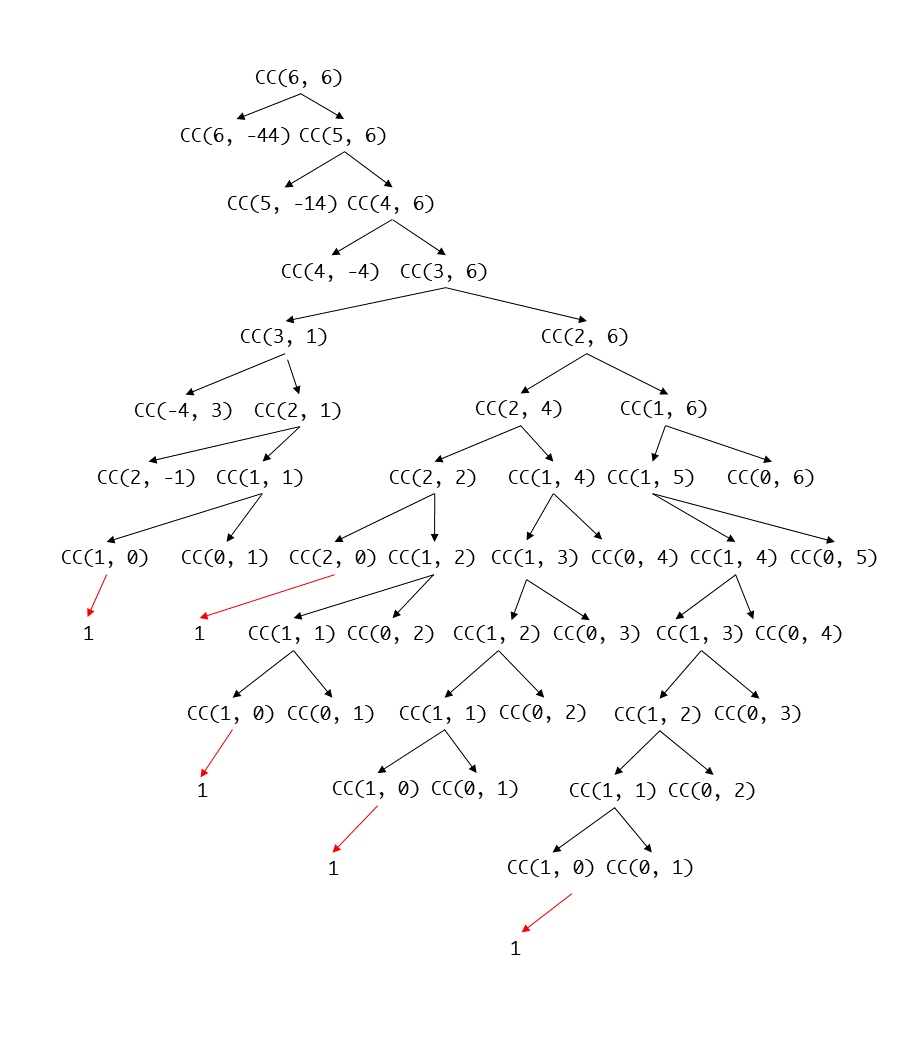Fenrier Lab

# 算法题：零钱兑换

### 问题描述与分析

1. 一部分是使用 $$k-1$$ 种纸币兑换 $$w$$ 元钱的方法数量 $$N_{k-1,w}$$
2. 另一部分用 $$k$$ 种纸币兑换成 $$w-v_k$$ 这么多钱的方法数量 $$N_{k, w-v_k}$$，之所以减去 $$v_k$$ 是为了留一个空缺，用 $$v_k$$ 来填充，保证此方法至少可以包含一张 $$v_k$$ 面额的。

$N_{k, w} = N_{k,w-v_k} + N_{k-1, w}$

$N_{k, w-v_k} = N_{k, w-v_{k}-v_{k-1}} + N_{k-1, w - v_k}$

$N_{k-1,w} = N_{k-1,w-v_k} + N_{k-2,w}$

1. 使用 0 种纸币来兑换 1 元钱，数量为 $$N_{0,1}$$
2. 使用 1 元纸币来兑换 0 元钱，数量为 $$N_{1,0}$$

$N_{1,1} = N_{0,1} +N_{1,0} = 1$

1. 当纸币数量不止一种，但是可兑换金额却等于 0 的时候，兑换方式也只有 1 种，那就是不使用任何纸币，即 $$N_{x, 0} = 1,1\le x\le k$$ 。
2. 而当可兑换金额小于零时，则不存在任何兑换方式，即 $$N_{x,y} = 0, y <0$$ 。
3. 并且当无纸币可用时，也不存在任何兑换方式，即 $$N_{0,y}=0$$ 。

### 代码实现

//version 0.1
public class CoinChange {
//纸币面额数组
private int[] v;

public CoinChange(int[] v) {
this.v = v;
}

public int countingChange(int w){
return countingChange(v.length, w);
}

private int countingChange(int k, int w) {
if(k <= 0 || w < 0) {
return 0;
}
if(w == 0) {
return 1;
}
return countingChange(k - 1, w) + countingChange(k,w - v[k-1]);
}

public static void main(String[] args) {
int[] v = {1, 2, 5, 10, 20, 50};
int W = 100;

int count = new CoinChange(v).countingChange(W);
System.out.println(count);
}
}


### 重构 1//version 0.2
public class CoinChange {

private int[] v;

public CoinChange(int[] v) {
this.v = v;
}

public int countingChange(int w) {
//申明中间过程缓存数组，并将所有值置为 -1，以便检查是否已有缓存值
int[][] cache = new int[v.length][w + 1];
for (int i = 0; i < cache.length; i++) {
Arrays.fill(cache[i], -1);
}
return countingChange(v.length, w, cache);
}
private int countingChange(int index, int w, int[][] cache) {
if(k <= 0 || w < 0) {
return 0;
}
if(w == 0) {
return 1;
}
int c1, c2;
//如果发现要计算的值已被缓存，那么直接读取即可，否则进行计算，并且将结果缓存到 cache 数组
if(cache[k-1][w] != -1) {
c1 = cache[k-1][w];
}else{
c1 = countingChange(k - 1, w, cache);
cache[k - 1][w] = c1;
}
if(w - v[k-1] >= 0 && cache[k][w - v[k-1]] != -1) {
c2 = cache[k][w - v[k-1]];
}else{
c2 = countingChange(k,w - v[k-1], cache);
if(w - v[k-1] >= 0) {
cache[k][w - v[k-1]] = c2;
}
}
cache[k][w] = c1 + c2;
return c1 + c2;
}

public static void main(String[] args) {
int[] v = {1, 2, 5, 10, 20, 50};
int W = 100;

int count = new CoinChange(v).countingChange(W);
System.out.println(count);
}
}


### 重构 2

cache[k][w] = cache[k][w - v[k-1]] + cache[k-1][w];


//version 0.3
public class CoinChange {

private int[] v;
public CoinChange(int[] v) {
this.v = v;
}

public int countingChange(int w) {
int[][] cache = new int[v.length + 1][w + 1];
//初始化 cache
for (int i = 1; i < cache.length; i++) {
cache[i] = 1;
}
for (int i = 0; i < cache.length; i++) {
cache[i] = 0;
}

for (int i = 1; i < v.length + 1; i++) {
for (int j = 1; j < w + 1; j++) {
int index = j - v[i - 1];
int temp;
if(index < 0) {
temp = 0;
}else {
temp = cache[i][index];
}
cache[i][j] = temp + cache[i - 1][j];
}
}
return cache[v.length][w];
}

public static void main(String[] args) {
int[] v = {1, 2, 5, 10, 20, 50};
int W = 100;

int count = new CoinChange(v).countingChange(W);
System.out.println(count);
}



cache[i][j] = temp + cache[i - 1][j];